# NABARD Manager Reasoning Mock Test 4

## 20 Questions MCQ Test NABARD Manager - Mock Tests & Previous Year Papers | NABARD Manager Reasoning Mock Test 4

Description
Attempt NABARD Manager Reasoning Mock Test 4 | 20 questions in 12 minutes | Mock test for Banking Exams preparation | Free important questions MCQ to study NABARD Manager - Mock Tests & Previous Year Papers for Banking Exams Exam | Download free PDF with solutions
QUESTION: 1

### Given question below consists of a question and two statements numbered I and II. You have to decide whether the data provided in the statements are sufficient to answer the question. How many minutes does the clock lose a day? Statements: I. The clock reads 6 : 00 when it is really 5 : 48 II. The clock is 40 seconds fast each hour.

Solution:

Statement I: Clock is fast by 12 minutes each hour
Statement II: Clock is fast by 2/3 of a minutes i.e. 40 second
Hence, the question can be answered by using either of the statements

QUESTION: 2

Solution:
QUESTION: 3

### Cars are safer than planes. Fifty percent of plane accidents result in death, while only one percent of car accidents result in death. Which of the following, if true, would most seriously weaken the argument above?

Solution:
QUESTION: 4

There are five different houses, A to E in a row. A is to the right of B and E is to the left of C and right of A. B is to the right of D.Which of the houses is in the middle?

Solution:
QUESTION: 5

A customer returning defective merchandise should be given an immediate refund if the merchandise was defective when purchased and was not on sale; if the customer is returning merchandise that is not defective or was damaged by customer negligence or customer abuse, the customer should be referred to the manager.
Which one of the following judgments conforms most closely to the principle stated above?

Solution:
QUESTION: 6
What does 'come' represent in a code language ?
I. 'pit na tac' means 'come and go' in that code language.
II. 'ja ta da' means 'you are good' in that code language.
III. 'na da rac' means 'you can come' in that code language.
The question given above has a problem and three statements giving certain information. you have to decide if the information given in the statements is sufficient for answering the problem. Indicate your answer as
Solution:
QUESTION: 7

When I am elected, I will work towards effecting those changes for which I have been fighting all these years. We will work together to do away with the bureaucratic bogs which have existed ever since my opponent took office. Everyone of you knows what I stand for; I invite my opponent to ...
For completion of the above statement choose the best option:

Solution:
QUESTION: 8

In the following questions, the symbols αα,ββ,δδ,γγ and ηη are used with the following .
'P αα Q' means 'P greater than Q'
'P ββ Q' means 'P is either greater than or equal to Q'.
P δδ Q means P is equal to Q.
P γγ Q means P is smaller then Q.
P ηη Q means P is either smaller than or equal to Q.
Now in each of the following questions assuming the given statements to be true, find out which of the two conclusions I and II given below them is/are definitely true?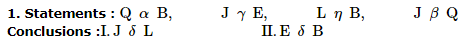Solution: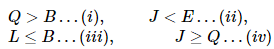By an intelligent observation we find there is no sign of δδ in any equation given in the statement. Hence, we can conclude within a second that both I and II are not true.

QUESTION: 9

In the following questions, the symbols αα,ββ,δδ,γγ and ηη are used with the following .
'P αα Q' means 'P greater than Q'
'P ββ Q' means 'P is either greater than or equal to Q'.
P δδ Q means P is equal to Q.
P γγ Q means P is smaller then Q.
P ηη Q means P is either smaller than or equal to Q.
Now in each of the following questions assuming the given statements to be true, find out which of the two conclusions I and II given below them is/are definitely true?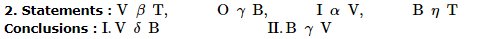Solution: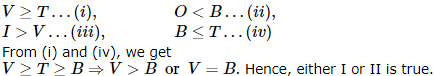QUESTION: 10

In the following questions, the symbols αα,ββ,δδ,γγ and ηη are used with the following .
'P αα Q' means 'P greater than Q'
'P ββ Q' means 'P is either greater than or equal to Q'.
P δδ Q means P is equal to Q.
P γγ Q means P is smaller then Q.
P ηη Q means P is either smaller than or equal to Q.
Now in each of the following questions assuming the given statements to be true, find out which of the two conclusions I and II given below them is/are definitely true?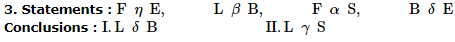Solution:

Again, by an intuitie look we come to know L is not equal to nay element in the given equations in the statements. Hence, I is not true. Again, as you have in our Magiical Book Series on Analytical Reasoning by MK Pandey, Tip 8 say 'If a term was not less than (or, less than or equal to') any other term in the given statements, then it can't be less than (or, "less than or equal to") any term in a conclusion. (Such a conclusion is definitely false) Thus II is not true.

QUESTION: 11

Ten persons P, Q, R, S, T, U, V, W, X and Y are sitting in two rows with five persons in each row in such a way that one person in the first row sits exactly opposite and facing a person in the second row. Members of the first row are facing North.
Q sits in the first row to the immediate right of W who sits exactly opposite of S. R is the extreme end of the second row and is second to the left of S. P is to the immediate right of S and exactly opposite to U. V sits exactly opposite to T who is st one of the ends of the second row. Y does not sits at the end.

Q. Which of the following pairs of persons are sitting at the two ends of the first row?

Solution: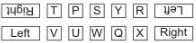QUESTION: 12

Ten persons P, Q, R, S, T, U, V, W, X and Y are sitting in two rows with five persons in each row in such a way that one person in the first row sits exactly opposite and facing a person in the second row. Members of the first row are facing North.
Q sits in the first row to the immediate right of W who sits exactly opposite of S. R is the extreme end of the second row and is second to the left of S. P is to the immediate right of S and exactly opposite to U. V sits exactly opposite to T who is st one of the ends of the second row. Y does not sits at the end.

Q. Who is second to the left of Q?

Solution:QUESTION: 13

Ten persons P, Q, R, S, T, U, V, W, X and Y are sitting in two rows with five persons in each row in such a way that one person in the first row sits exactly opposite and facing a person in the second row. Members of the first row are facing North.
Q sits in the first row to the immediate right of W who sits exactly opposite of S. R is the extreme end of the second row and is second to the left of S. P is to the immediate right of S and exactly opposite to U. V sits exactly opposite to T who is st one of the ends of the second row. Y does not sits at the end.

Q. Who is third to the left of T?

Solution:QUESTION: 14

Study the given information carefully and answer the given questions:
Twenty students are standing in a straight line facing north. Rina is standing sixth from the left end. There are only three students between Rina ans Shweta. Radha is standing exactly between Shweta and Rina. Tina is standing sixth to the right of Radha. Anita is standing fourth from the right end of the line. There are more than four students between Rina and Tina.

Q. How many people are standing between Anita and Tina?

Solution: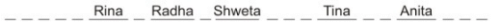QUESTION: 15

A word and number arrangement machine when given an input line of words and numbers rearranges them following a particular rule in each step. The following is an illustration of input and rearrangement.
Input : gone 93 over 46 84 now for 31
Step I : 31 gone 93 over 46 84 now for
Step II : 31 over gone 93 46 84 now for
Step III : 31 over 46 gone 93 84 now for
Step IV : 31 over 46 now gone 93 84 for
Step V : 31 over 46 now 84 gone 93 for
and Step V is the last step of the rearrangement of the above input. As per the rules followed in the above steps, find out in each of the following questions the appropriate step for the given input.

Q. Step III of an Input: 15 window 2993 86 sail tower buy Which of the following will be step VI?

Solution:
QUESTION: 16

A word and number arrangement machine when given an input line of words and numbers rearranges them following a particular rule in each step. The following is an illustration of input and rearrangement.
Input : gone 93 over 46 84 now for 31
Step I : 31 gone 93 over 46 84 now for
Step II : 31 over gone 93 46 84 now for
Step III : 31 over 46 gone 93 84 now for
Step IV : 31 over 46 now gone 93 84 for
Step V : 31 over 46 now 84 gone 93 for
and Step V is the last step of the rearrangement of the above input. As per the rules followed in the above steps, find out in each of the following questions the appropriate step for the given input.

Q. Input: station hurry 39 67 all men 86 59 How many steps will be required to complete the rearrangement?

Solution:
QUESTION: 17

A word and number arrangement machine when given an input line of words and numbers rearranges them following a particular rule in each step. The following is an illustration of input and rearrangement.
Input : gone 93 over 46 84 now for 31
Step I : 31 gone 93 over 46 84 now for
Step II : 31 over gone 93 46 84 now for
Step III : 31 over 46 gone 93 84 now for
Step IV : 31 over 46 now gone 93 84 for
Step V : 31 over 46 now 84 gone 93 for
and Step V is the last step of the rearrangement of the above input. As per the rules followed in the above steps, find out in each of the following questions the appropriate step for the given input.

Q. Step II of an input is : 49 zone car battery 56 87 71 down Which of the following is definitely the input?

Solution:
QUESTION: 18

Below are the statements followed by two conclusions numbered I and II. You have to consider the statements and the following conclusions and decide which of the conclusion(s) follows the statement(s).

Statements:
a.All jugs are plates.
b. All plates are cups.
c.All cups are bottles.
Conclusions :
I. Some bottles are jugs.
II. All plates are bottles.

Solution:
QUESTION: 19

Below are the statements followed by two conclusions numbered I and II. You have to consider the statements and the following conclusions and decide which of the conclusion(s) follows the statement(s).

Statements:
a. Some jungles are mirrors.
b. All mirrors are houses.
Conclusions :
II. All jungles are houses.

Solution:
QUESTION: 20

Below are the statements followed by two conclusions numbered I and II. You have to consider the statements and the following conclusions and decide which of the conclusion(s) follows the statement(s).

Statements:
a. All pigeons are trees.
b. Some trees are channels.Use Code STAYHOME200 and get INR 200 additional OFF Use Coupon Code